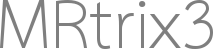# Sampling FOD maxima

Hello,

is there a way to sample from an FOD without having to touch the code?

Basically I want to know the orientation with the largest angle (compared to the mean/main orientation of the FOD) you could sample from the FOD (talking about a FOD with only one peak).

I guess a different way would be to calculate the “width” or dispersion of the FOD lobe. You could do this using fod2fixel, but this only gives you a relative dispersion. As far as I know you can not get the maximum angle from his relative dispersion. You would need a quantitative dispersion I guess.

I wonder if there is a way to do this without having to get into the mrtrix code base.

Thanks
Jakob

I think sh2amp will do what you need?

I will give this a try. Thank you!

Hey Jakob,

`sh2amp` will indeed be the key to this. Other than that, to pitch in to this particular one:

I think I’m getting what you’re after or might be thinking about; but just something to take into account: it might look like an FOD (with only one peak, or in any case) has pretty narrow lobes due to how it’s rendered with the radius scaling type of visualisation we’re used to looking at (the “3D” FODs we all look at in the viewer), but that’s sometimes a bit deceiving. The angle to which you can still sample a positive amplitude is pretty wide actually. Also, beyond that, the amplitude will not hit a “constant” zero for the rest of the angular domain. Instead, you’ll get a Gibbs effect: it’ll briefly dip below zero and start to then Gibbs-style over and undershoot around zero (at best). This matters for what you’re trying to measure / extract here: `sh2amp` only gives you discrete samples for a predefined set of orientations, so it’s hard to get from that the exact extent to which the “lobe stretches”.
What I’d recommend to get something like this more robustly, is to basically apply a small amplitude threshold, just like we do to segment fixels or perform tractography: essentially within those commands/algorithms, the threshold is the thing that says “here’s where the lobe ends, the rest might just be noise or something else undesirable”. So at a high level, to get the thing you’re after, you could do something along the lines of:

• Get the peaks (and thus their orientation) itself via `sh2peaks`.
• Sample the FOD via `sh2amp` for a relatively dense set of orientations.
• Then threshold the output of this to the small amplitude I mentioned. Use a similar value you’d use for fixel segmentation or tractography, e.g. 0.06 or something similar.
• After this thresholding, find the orientation among those that survived the threshold (i.e. the set of orientations “part of” the lobe) which has the largest angle with the peak you got earlier from `sh2peaks`.
• This is the angle you’re after (up to a limited precision, which relates directly to the density of the FOD sampling you used for `sh2amp`).

If you can assume up front that the FODs for which you do this only have one large main peak (and the next peak in line is basically very small, or just noise or Gibbs effect related; and thus falls below the applied threshold in the above process), this should be very robust.

Cheers,
Thijs

You could do this using fod2fixel, but this only gives you a relative dispersion.

The existing dispersion parameter is literally just the integral divided by the peak value; so it’s pretty rough.

You would need a quantitative dispersion I guess.

I gave this a go a while back, but haven’t had a chance to revisit. The existing algorithm in `fod2fixel` was actually intended to be only the first step in this algorithm, but it proved to be adequate in its own right for almost all applications.

Thanks for all the answers. I got it working as intended using `sh2amp`. Getting the conversion from spherical coordinates to cartesian coordinates right was a bit tricky. In case anybody will need this in the future here is the python code that worked for me:

``````def sph2cart(az, el, r=1):
r_sin_el = r * np.sin(el)
x = r_sin_el * np.cos(az)
y = r_sin_el * np.sin(az)
z = r * np.cos(el)
return x, y, z

def cart2sph(x, y, z):
r = np.sqrt(x*x + y*y + z*z)
az = np.arctan2(y, x)
el = np.arccos(z / r)
return az, el, r
``````
1 Like